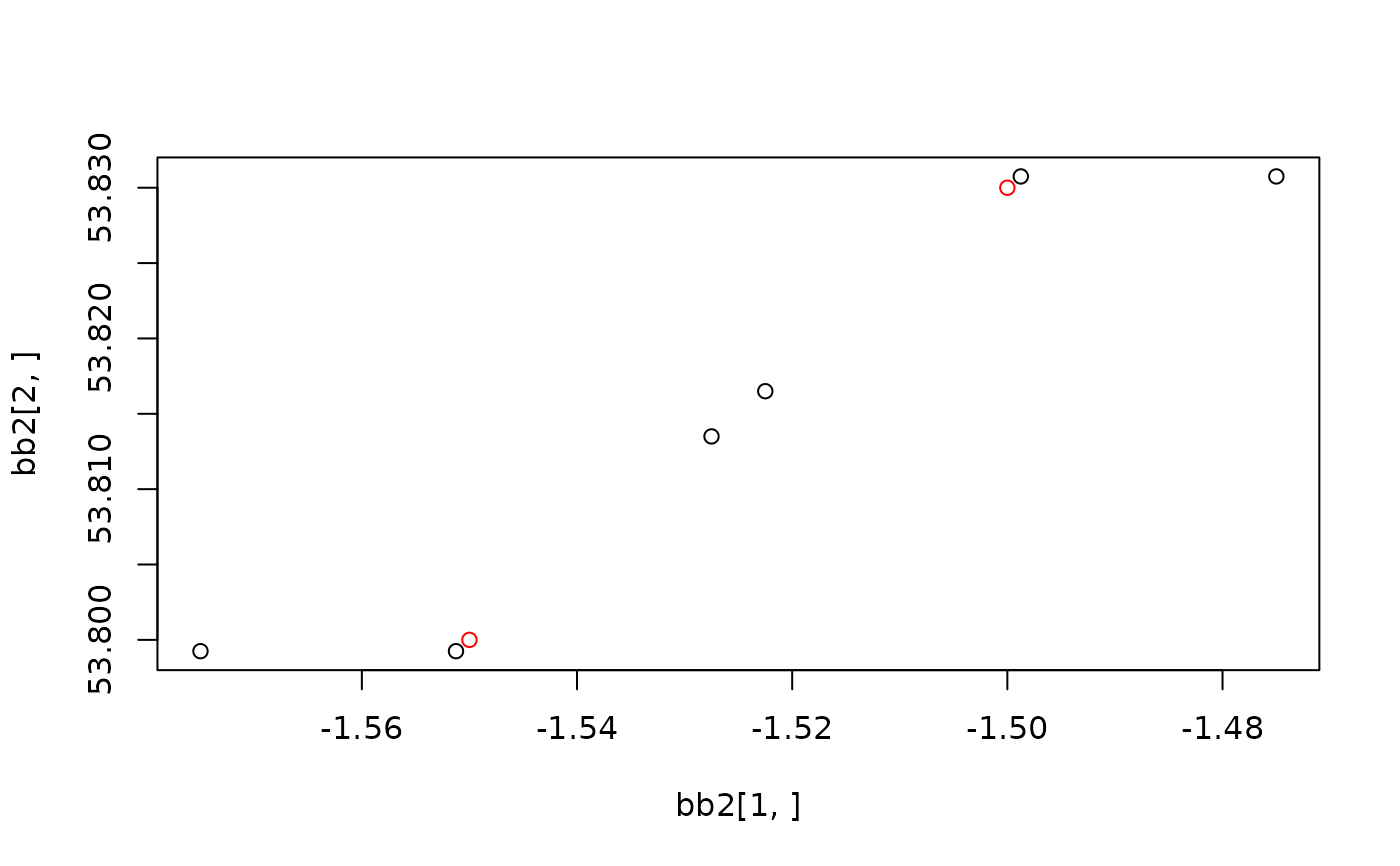Takes a bounding box as an input and outputs a bounding box of a different size, centred at the same point.

## Usage

``bbox_scale(bb, scale_factor)``

## Arguments

bb

Bounding box object

scale_factor

Numeric vector determining how much the bounding box will grow or shrink. Two numbers refer to extending the bounding box in x and y dimensions, respectively. If the value is 1, the output size will be the same as the input.

Other geo: `geo_bb_matrix()`, `geo_bb()`, `quadrant()`

## Examples

``````bb <- matrix(c(-1.55, 53.80, -1.50, 53.83), nrow = 2)
bb1 <- bbox_scale(bb, scale_factor = 1.05)
bb2 <- bbox_scale(bb, scale_factor = c(2, 1.05))
bb3 <- bbox_scale(bb, 0.1)
plot(x = bb2[1, ], y = bb2[2, ])
points(bb1[1, ], bb1[2, ])
points(bb3[1, ], bb3[2, ])
points(bb[1, ], bb[2, ], col = "red")``````